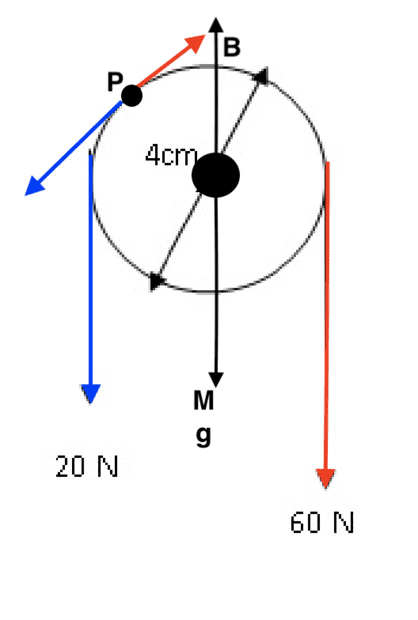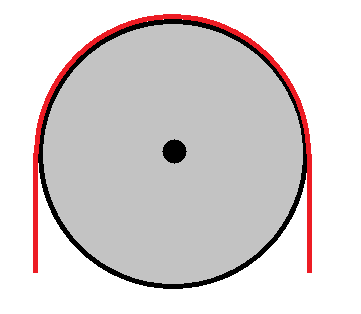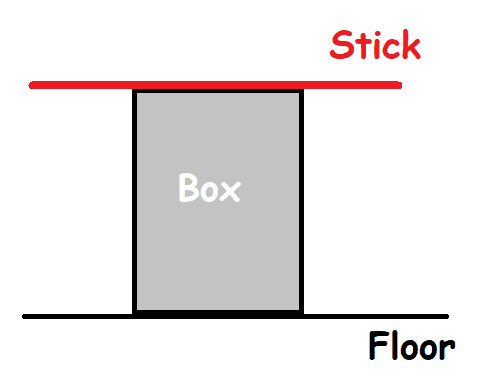# Translational force of a pulley

• I
annamal
Below is a pulley with mass and a string around it where the tension of one end of the string is 20 N and the tension of the other end of the string is 60 N. I know there is a net torque due to the differing tensions of the string, so I am wondering what the translational forces are on the pulley. We have a force B of the screw holding the pulley up against the weight of the pulley Mg. So would the translational force in the y direction of the pulley be
-20 - 60 - Mg + B = 0?

If so, I am wondering how to translationally (not rotationally) take into account forces on other points of the pulley like at point P. For example, due to the tension in the string around the pulley, there is a 20 N force in the ##-\vec i + -\vec j## direction and a 60 N force in the ##\vec i + \vec j## direction. Is that correct? Because it seems like I am adding extra force on the pulley.•Delta2

Have you considered the "triangle of forces" resolved about the centre of the pulley?

annamal
Have you considered the "triangle of forces" resolved about the centre of the pulley?
How do you draw the triangle?

How do you draw the triangle?
That would require that you have both x and y coordinates for the applied forces.
But I don't think you yet have enough data to resolve the problem.

An alternative would be to treat the pulley as a diameter-long beam-balance, and find the position of the fulcrum on that diameter. The fulcrum will be at half the radius, on the RHS. Would the pulley swing sideways to place its centre there, with B being no longer vertical?
Again, insufficient information.

•Lnewqban
annamal
That would require that you have both x and y coordinates for the applied forces.
But I don't think you yet have enough data to resolve the problem.

An alternative would be to treat the pulley as a diameter-long beam-balance, and find the position of the fulcrum on that diameter. The fulcrum will be at half the radius, on the RHS. Would the pulley swing sideways to place its centre there, with B being no longer vertical?
Again, insufficient information.
Would just -20 - 60 - Mg + B = 0 be enough?

Homework Helper
Gold Member
Well if you going to consider the forces at all the points along the circumference of the pulley between the rope and the pulley, then you have to do some sort of treatment similar to what we do in beam theory. Every ##dl## of rope that is in contact with the pulley along the circumference applies a force ##d\vec{T}## to the pulley which is tangential to the pulley at that point, the total force would be $$\vec{F}=\int_0^{\pi} d\vec{T}$$. After we calculate the integral we 'll find that the vertical component of ##\vec{F}## is ##60+20## and there might be also horizontal component.

So yeah the main flaw in what you did is that you assumed that the forces at P are finite, while in fact are infinitesimal.

annamal
Well if you going to consider the forces at all the points along the circumference of the pulley between the rope and the pulley, then you have to do some sort of treatment similar to what we do in beam theory. Every ##dl## of rope that is in contact with the pulley along the circumference applies a force ##d\vec{T}## to the pulley which is tangential to the pulley at that point, the total force would be $$\vec{F}=\int_0^{\pi} d\vec{T}$$. After we calculate the integral we 'll find that the vertical component of ##\vec{F}## is ##60+20## and there might be also horizontal component.

So yeah the main flaw in what you did is that you assumed that the forces at P are finite, while in fact are infinitesimal.
I just want the translational force of the pulley and am not sure if to calculate the translation force of the pulley I would have to consider all the forces at all the points along the circumference

Homework Helper
Gold Member
I just want the translational force of the pulley and am not sure if to calculate the translation force of the pulley I would have to consider all the forces at all the points along the circumference
Yes that's the translation force of the pulley, you have to consider all the forces at all the points along the upper circumference of the pulley. The total torque would be $$\vec{M}=\int_0^{\pi} \vec{r}\times d\vec{T}$$ where ##\vec{r}## the vector along the radius of the pulley at the current point. It has constant magnitude equal to the radius of the pulley but its direction changes so that it always normal to the element ##dl##.

annamal
Yes that's the translation force of the pulley, you have to consider all the forces at all the points along the upper circumference of the pulley. The total torque would be $$\vec{M}=\int_0^{\pi} \vec{r}\times d\vec{T}$$ where ##\vec{r}## the vector along the radius of the pulley at the current point. It has constant magnitude equal to the radius of the pulley but its direction changes so that it always normal to the element ##dl##.
If that's the total torque how come a lot of the videos show torque in my example as 20r - 60r where r is the radius of the pulley?

Source:

•Delta2
Homework Helper
Gold Member
If that's the total torque how come a lot of the videos show torque in my example as 20r - 60r where r is the radius of the pulley?

Source:

My integral will give similar result for torque. As an excersice in vector calculus take as t$$d\vec{T}=20d\vec{l}=20(-\sin\phi\hat xdx+\cos\phi\hat ydy) \text{for}, 0<\phi<\frac{\pi}{2}$$ and $$d\vec{T}=-60d\vec{l}=-60(-\sin\phi\hat xdx+\cos\phi\hat ydy)\text{for}, \frac{\pi}{2}<\phi<\pi$$
and calculate the torque integral and see what you get.

annamal
My integral will give similar result for torque. As an excersice in vector calculus take as t$$d\vec{T}=20d\vec{l}=20(-\sin\phi\hat xdx+\cos\phi\hat ydy) \text{for}, 0<\phi<\frac{\pi}{2}$$ and $$d\vec{T}=-60d\vec{l}=-60(-\sin\phi\hat xdx+\cos\phi\hat ydy)\text{for}, \frac{\pi}{2}<\phi<\pi$$
and calculate the torque integral and see what you get.
I am wondering how come you are dividing the tensions into ##0<\phi<\frac{\pi}{2}## and ##
\frac{\pi}{2}<\phi<\pi##. I interpreted the tension being 20 N - 60 N at all infinitesimal points around the pulley.

Also I do not understand why we have to multiply by dl in ##d\vec{T}=20d\vec{l}##. Tension is just the force. Do you mean that is the ##d\tau## torque?

Instead of dx and dy in the equation, it should be ##d\phi##?

Also, it should be +60 not -60?

•Delta2
Homework Helper
Gold Member
Good questions! Hold on while I reply, it might take some time.

Homework Helper
Gold Member
Yes you can replace ##dx=-R\sin\phi d\phi ,dy=R\cos\phi d\phi##, those equations come form the polar coordinates equations ##x=R\cos\phi, y=R\sin\phi## where ##R## the radius of the pulley is considered constant.
It is -60 because if we take the upper midpoint of the circumference of the pulley, then the rope that is at left has tension tangential and pointing towards left (and down) while the rope that is at right has the tension pointing right (and down). That's why it is +20 for ##\phi<\pi/2## and -60 for ##\phi>\pi/2##.

We have to multiply by some infinitesimal tangential vector, I chose the infinitesimal arc length ##dl## but I did something wrong after all I should have divided the 20 (and 60) by the length of circumference that corresponds to angle ##\pi/2## that is ##\frac{\pi}{2}R##, so it should be e.g. ##d\vec{T}=\frac{20}{\frac{\pi}{2}R}d\vec{l}##

And to calculate the torque is fairly easy actually cause we know that the vector ##\vec{r}## which has magnitude R (radius of pulley) is always perpendicular to ##d\vec{l}## so it will be $$\vec{r}\times d\vec{T}=|\vec{r}||d\vec{T}|\sin \frac{\pi}{2}=R\frac{20}{\frac{\pi}{2}R}|d\vec{l}|$$ so the integral reduces to calculating the total arc length weighted by some factor.

What might be a good vector calculus excersise will be to calculate $$\int_0^{\pi}d\vec{T}$$.

Homework Helper
What is a "translational force"? How is it different from a "force"?

•malawi_glenn and Delta2
annamal
What is a "translational force"? How is it different from a "force"?
Translational is the force in the x and y directions.

Homework Helper
Then in what direction should a force be so it is not translational?

annamal
Then in what direction should a force be so it is not translational?
Not translational is rotational force

Homework Helper
Gold Member
2022 Award
@annamal Consider this example, a homogenous string (red color) with mass m is placed on the pulley (cylinder) with mass M, so that the system is in rest, see figure below.What is the magnitude and direction which the pivot is exerting on the cylinder?

Isn't it just (M+m)g straight up?

annamal
Yes you can replace ##dx=-R\sin\phi d\phi ,dy=R\cos\phi d\phi##, those equations come form the polar coordinates equations ##x=R\cos\phi, y=R\sin\phi## where ##R## the radius of the pulley is considered constant.
It is -60 because if we take the upper midpoint of the circumference of the pulley, then the rope that is at left has tension tangential and pointing towards left (and down) while the rope that is at right has the tension pointing right (and down). That's why it is +20 for ##\phi<\pi/2## and -60 for ##\phi>\pi/2##.

We have to multiply by some infinitesimal tangential vector, I chose the infinitesimal arc length ##dl## but I did something wrong after all I should have divided the 20 (and 60) by the length of circumference that corresponds to angle ##\pi/2## that is ##\frac{\pi}{2}R##, so it should be e.g. ##d\vec{T}=\frac{20}{\frac{\pi}{2}R}d\vec{l}##

And to calculate the torque is fairly easy actually cause we know that the vector ##\vec{r}## which has magnitude R (radius of pulley) is always perpendicular to ##d\vec{l}## so it will be $$\vec{r}\times d\vec{T}=|\vec{r}||d\vec{T}|\sin \frac{\pi}{2}=R\frac{20}{\frac{\pi}{2}R}|d\vec{l}|$$ so the integral reduces to calculating the total arc length weighted by some factor.

What might be a good vector calculus excersise will be to calculate $$\int_0^{\pi}d\vec{T}$$.
What about "I am wondering how come you are dividing the tensions into 0<ϕ<π/2 and π/2<ϕ<π. I interpreted the tension being 20 N - 60 N at all infinitesimal points around the pulley."

So is ##dl = -R\sin\phi d\phi \vec i + R\cos\phi d\phi \vec j##
or ##-\sin\phi\hat xdx+\cos\phi\hat ydy = (-\sin\phi)(-R\sin\phi d\phi)\hat x+(\cos\phi)(R\cos\phi d\phi)\hat y##?

annamal
@annamal Consider this example, a homogenous string (red color) with mass m is placed on the pulley (cylinder) with mass M, so that the system is in rest, see figure below.
View attachment 303572
What is the magnitude and direction which the pivot is exerting on the cylinder?

Isn't it just (M+m)g straight up?
So with weights on either end of the red string, it would be (M+m + Weights)g?

Homework Helper
Gold Member
2022 Award
So with weights on either end of the red string, it would be (M+m + Weights)g?

There is no difference between increasing the weight of the string than hanging on some weights.

Because the pulley (cylinder) is in equilibrium, the net force on it must equate (vectorially) to zero.

Consider this too. A box with mass M is placed on the floor. On top of the box, a stick (red) with mass m is placed. What is the force on the box from the floor? Isn't it just (M + m)g upwards?Now place some weights on the stick, what is the force on the box from the floor now?

Homework Helper
Gold Member
What about "I am wondering how come you are dividing the tensions into 0<ϕ<π/2 and π/2<ϕ<π. I interpreted the tension being 20 N - 60 N at all infinitesimal points around the pulley."

So is ##dl = -R\sin\phi d\phi \vec i + R\cos\phi d\phi \vec j##
or ##-\sin\phi\hat xdx+\cos\phi\hat ydy = (-\sin\phi)(-R\sin\phi d\phi)\hat x+(\cos\phi)(R\cos\phi d\phi)\hat y##?
Sorry seems I messed up somewhere , it is indeed $$d\vec{l}=-R\sin\phi d\phi \hat i+ R\cos\phi d\phi \hat j$$

I told you why I divide the two regions of ##\phi##, it is because left and right of ##\phi=\pi/2## the tension changes direction from left to right.

Homework Helper
Not translational is rotational force
You are just making up names. But you are not able to define what these names mean.

Last edited:
•malawi_glenn
Homework Helper
Gold Member
A force can have either or both rotational and translational effect. E.g. when a ball rolls down an incline the force of friction reduces the translational acceleration but increases the rotational acceleration.

Homework Helper
The tension in the rope changes gradually from 20 N to 60 N.
For each section of rope there are two forces in opposite directions but these are not 20N and 60 N but the values of the tensions at that point. If you take a small element of rope, with angular size ##d \theta ##, the forces produced by the tension are ## T(\theta) ## and ##T(\theta +d\theta)## acting along the tangent and in (almost) opposite directions. This apply to any segment of the rope. There is no point where the tension suddenly changes direction. The tension does not even have a direction like a vector. The forces produced by the tension act like described above on any segment of rope bu the tension does not have a direction at a given point.

Homework Helper
Gold Member
2022 Award
Is a translational force a force that does not supply torque w.r.t. CM? Is a rotational force a a force that does not accelerate CM linearly? Never seen those concepts before.

Homework Helper
Is a translational force a force that does not supply torque w.r.t. CM? Is a rotational force a a force that does not accelerate CM linearly? Never seen those concepts before.
Possibly you did not see them because they are not useful. You can define these names as you say but what is the point? Looking on Google, I see some texts where they use the term "translational force" as opposite to "torque". Which is an useless specification because the torque is not another type of force but a completely different quantity. So there is no danger of confusing "force" with "torque". But it may be an useful distinction in mechanical engineering where they sometime use torque without reference to a force (or couple of forces) producing that torque.

•malawi_glenn and Delta2
Homework Helper
Gold Member
But it may be an useful distinction in mechanical engineering where they sometime use torque without reference to a force (or couple of forces) producing that torque.
Yes it also seems to me that this terminology is from mechanical engineering.